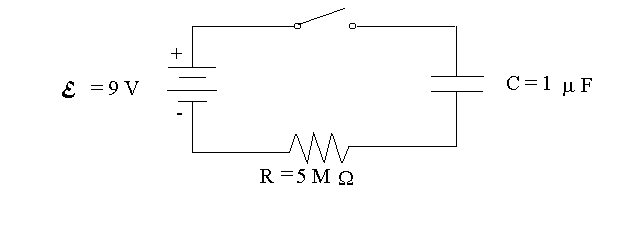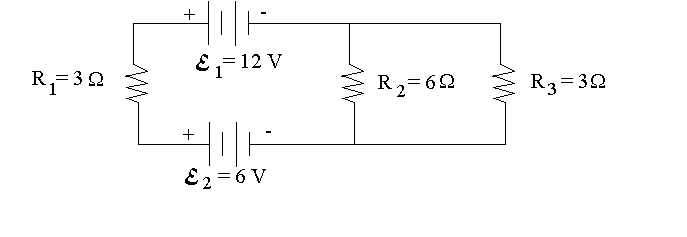First Name: ___________________ Last Name: ____________________ Section: _________

February 21, 1997 Physics 208

Exam 1

Print your name and section clearly on all five pages. (If you do not know your section number, write your TAs name.) Show all work in the space immediately below each problem. Your final answer must be placed in the box provided. Problems will be graded on reasoning and intermediate steps as well as on the final answer. Be sure to include units wherever necessary, and the direction of vectors. Each problem is worth 25 points. In doing the problems, try to be neat. Check your answers to see that they have the correct dimensions (units) and are the right order of magnitude. You are allowed one 8½ x 11" sheet of notes and no other references. The exam lasts exactly 50 minutes.

(Do not write below)

SCORE:

Problem 1: __________

Problem 2: __________

Problem 3: __________

Problem 4: __________

TOTAL: ___________

First Name: ___________________ Last Name: ____________________ Section: _________

1. An insulated sphere with radius a = 20 cm has a charge of Q = 10 µC distributed uniformly over its surface.

a. Calculate the electric field at a distance of 30 cm from the center of the sphere. (5 pts.)

b. Calculate the electric potential at the center of the sphere (relative to infinity). (5 pts.)

c. How much energy is required to remove an electron from the sphere and place it infinitely far away? (5 pts.)

d. What is the capacitance of the sphere relative to infinity? (5 pts.)

e. What is the total electric flux leaving the sphere? (5 pts.)

First Name: ___________________ Last Name: ____________________ Section: _________

1. Two parallel conducting plates, each with area A = 0.5 m2, are separated by a distance of d = 1 mm and have a voltage difference of V = 80 volts.

a. Calculate the magnitude of the electric field in the space between the plates. (5 pts.)

b. Calculate the capacitance of the plates. (5 pts.)

c. Calculate the charge on the positive plate. (5 pts.)

d. Calculate the energy stored in the capacitor. (5 pts.)

e. For how long would a current of 1 µA have to flow to fully discharge the capacitor? (5 pts.)

First Name: ___________________ Last Name: ____________________ Section: _________

1. In the circuit below, the capacitor is initially discharged when the switch is closed.a. What is the current in the resistor just after the switch is closed? (5 pts.)

b. Calculate the voltage across the capacitor 3 seconds after the switch is closed. (10 pts.)

c. How much total energy is supplied by the battery during the time required to fully charge the capacitor? (10 pts.)

First Name: ___________________ Last Name: ____________________ Section: _________

4. In the circuit below the batteries and resistors are ideal.a. Calculate the voltage across resistor R1. (10 pts.)

b. Calculate the power dissipated in resistor R1. (5 pts.)

c. Calculate the current in resistor R3. (10 pts.)